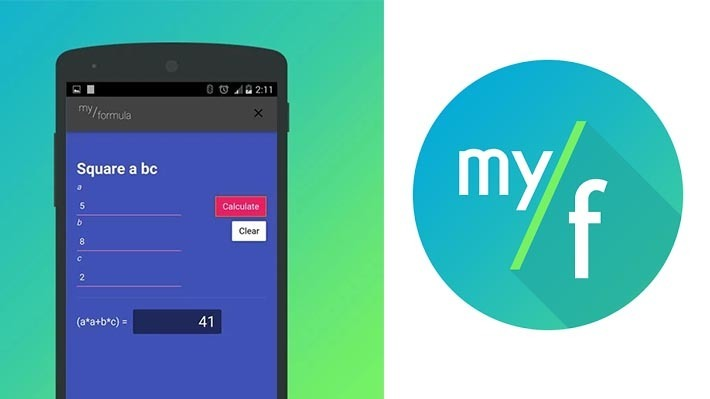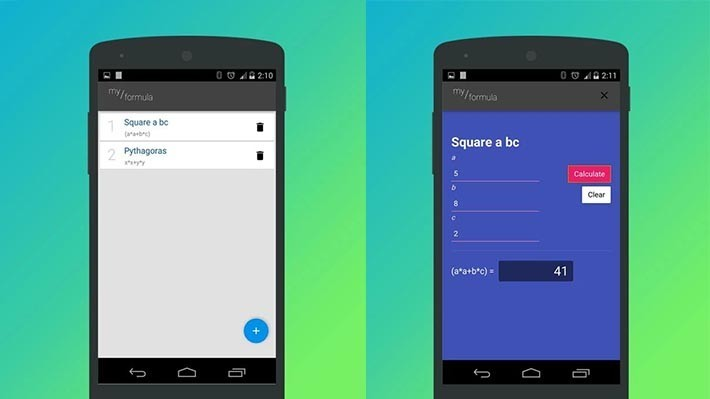Best daily deals

# myFormula - Indie app of the day

myFormula is a math application that allows you to create, save, and use complicated equations so you don't have to do them by hand. It's also totally free!
By
November 24, 2014## What is myFormula?

myFormula is an application that allows you to create and store formulas for easy reference. Think of it like a math cheat sheet that you carry around on your smartphone. It’s currently free in the Google Play Store with no in app purchases.

Here’s how the app works. You use it to create various mathematical equations. You can make things like the Pythagorean Theorem, Quadratics, and other math things that may take a lot of time to do by hand. Once you create the equations, you simply input the variables and the app will give you the answers. It’s a fairly simple app that you can use to create a lot of the more tedious equations.

It is an app for students and possibly even for teachers depending on what kind of math they teach. That means its real world applications are pretty limited. It’s also worth noting that as of right now, you can only use the basic math operators (plus, minus, multiply, divide), up to three variables (a, b, c), and parenthesis. That means there is a ceiling as to how complicated your equations can be unless you get really creative and do things like log functions by hand. and then use that equation in other equations.Overall, it’s not a bad application. Those taking algebra, geometry, and the other basic maths will definitely find this handy. Those who are into the more complicated stuff may find this app to be a tad too simple for the stuff you’re doing. Thankfully, it is free in the Play Store so you can check it out and see if it works for you!